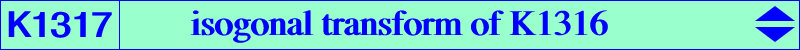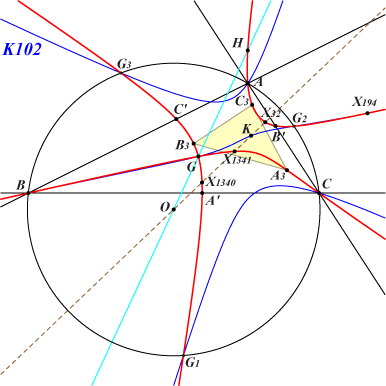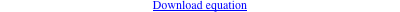too complicated to be written here. Click on the link to download a text file.X(2), X(4), X(32), X(194), X(1340), X(1341), X(1383), X(9463), X(14609), X(45096), X(52672), X(52765) A3, B3, C3 : vertices of the 3rd Brocard triangle vertices of the Grebe triangle i.e. points of K102 on (O) infinite points of pK(X6,X3734) Geometric properties :K1317 is the isogonal transform of K1316, the mid-cevian cubic MC(X6) as in CL069. K1317 is a nodal cubic with node X(2). The nodal tangents are parallel to the asymptotes of the circum-conic whose perspector is the barycentric product X(523) x X(7804). K1317 meets the sideline BC at A', on the line passing through X(32) and the A-vertex of the circumcevian triangle of X(2). B' and C' are defined likewise. The triangles A'B'C' and A3B3C3 are perspective at Z on the cubic. Z = b^2 c^2 (a^6 b^2+a^4 b^4+a^6 c^2+a^4 c^4-b^4 c^4) : : , SEARCH = -6.62143023941086, on the lines {2,308}, {4,6331}, {32,689}. If P = u : v : w ≠ G is a point, then Q is a point on K1317, where Q = a^2 (-a^2 (b^2 + c^2) + b^4 (w - u) / (v - w) + c^4 (u - v) / (v - w) ) : : .